# Bayesian recipes (3): Simple, efficient statistics for Normal distributions

### Contents

The simple Bayesian approach to Normal distributions lets you understand exactly what you can conclude from limited evidence, even if it's not very conclusive. And, as ever, it's easier to understand and produces good looking graphs.

This article explains some simple techniques that can be done using Excel. Of all the distributions I will cover in this series of articles, this one is perhaps the most worth writing about. In practice it is very easy to use but other explanations I have seen are maddeningly complicated and confusing. Even with the explanation below it is easy to get a bit confused as to which distribution you are doing so be patient and take the time to pay attention to details and try things out yourself with some data.

# Where these techniques can be used

These techniques can be used wherever you sample measurements and believe them to be Normally distributed, and what you want to understand is the true distribution of measurements for a large population or for a process producing population items.

With these criteria met, you then need at least two actual measurements, sampled at random, and you're in business. The sample could be selected by throwing dice, or using the the RAND() function in Excel, or just closing your eyes and dipping in.

With the techniques in this article you can enter each measurement, one at a time if you like, and immediately see the impact. This allows you to stop the moment you realise you have a clear enough picture.

Before you start considering the historical data, what do you know about the pattern of measurements? The measurements are going to be lots of different values. If you had data from many, many measurements you could create a histogram showing how often each measurement value occurs. What would it look like?

It's very possible that it would look like the Normal distribution, which is a curve described by a particular mathematical formula. The Normal distribution can have many different shapes depending on the values of two numbers that appear in its formula, known as the distribution's parameters. One parameter is its average and other is its spread. Here are some examples of Normal distributions with different averages and spreads.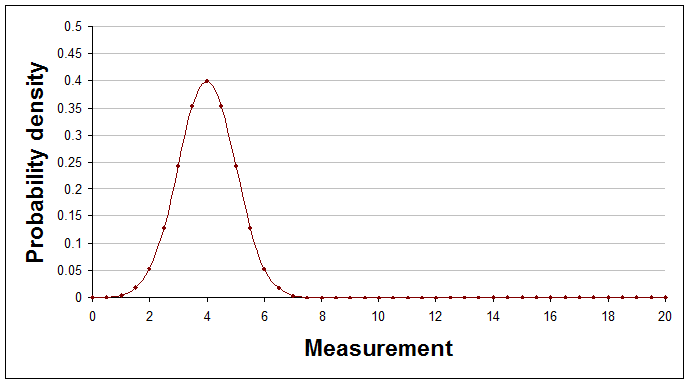Average of 4, spread (standard deviation) of 1.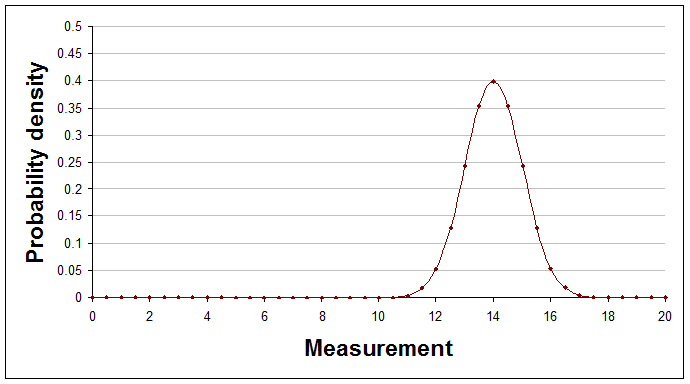Average of 14, spread (standard deviation) of 1.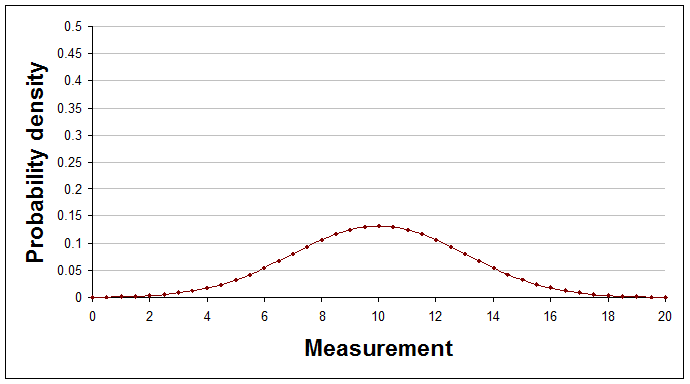Average of 10, spread (standard deviation) of 3.

If you have good reasons to think that the actual distribution, with a really large number of measurements, would be one of these Normal distributions then the real question is, what do you know about the average and spread of this particular curve?

You might think that you've no idea about the average and spread, and that they could just as well be any number at all, but is that reasonable? Probably you have some rough idea even before you consider any data.

Here's a sequence of pictures showing how your view of the average and spread of the Normal distribution would change after each result is learned and added to the analysis. The very first curve is thinly spread out, indicating that at the start the analyst chose to have a very open mind about the average and spread.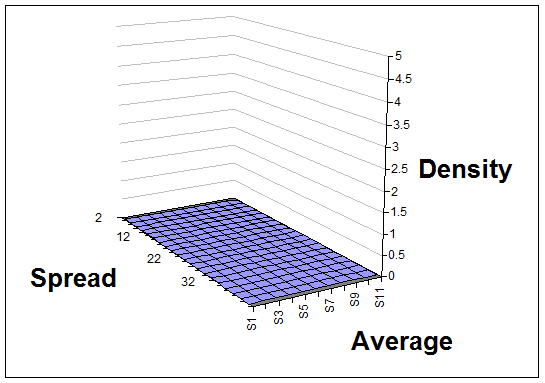With no data so far - just flat/undefined.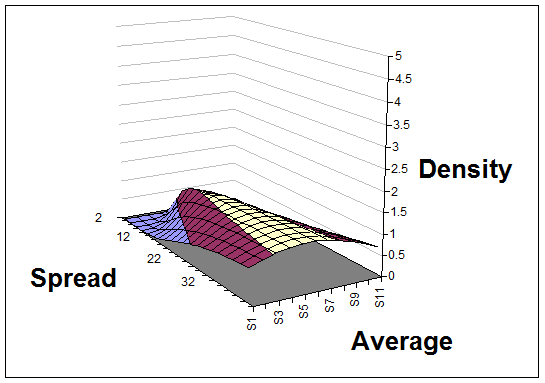After two data points (28 and 35) the spread could still be very large but a sense of the average is already beginning to show.After three data points (28, 35, and 42) the spread is now taking shape too.After seven data points (28, 35, 42, 43, 44, 50, and 51) the distribution of average and spread is really taking shape.

A quick look at the surface, as it evolves, gives you a sense of where the truth probably lies, and further calculations or additional graphs can answer questions like "How likely is it that the average measurement of the true distribution is more than 30, given the evidence we have so far?"

# The technicalities explained

Here's a quick explanation of the technicalities, with plain English translation.

Technical terms Explanation
The surfaces showing the mean and standard deviation of the Normal distribution are probability density functions. A probability density function shows how likely you think each combination of mean (i.e. average) and standard deviation (i.e. spread) is. Probability density functions never go negative, but they can reach high peaks. The volume under the surface is always exactly 1.
The surfaces represent normal-gamma distributions, having parameters mu (pronounced 'myoo'), kappa, alpha, and beta. The normal-gamma distribution is a mathematical formula that can describe many different shapes of surface depending on the values of four numbers used within it. These numbers are called parameters and the parameters for the normal-gamma distribution are usually called mu, kappa, alpha, and beta.
The normal-gamma distribution is a conjugate distribution for the normal likelihood function. The normal-gamma distribution being a 'conjugate' distribution just means that the surface before and after historical results are processed remains a normal-gamma distribution, but with modified parameter values. It is the crucial changes to the parameter values that cause the surface to change its shape. A normal likelihood function is just the type of the distribution of long run relative frequencies.

# Doing it in Excel

## Processing new results

Working out the surfaces for the distribution of the average and spread in response to new data requires simple arithmetic, but to simplify things further I have provided new Excel functions to do those calculations. If you want to produce graphs or answer questions about the surfaces and other curves then you need to use further Excel functions.

To process a sample of data (which could have one or more data points in it) you first need to calculate three numbers about the sample using the appropriate Excel functions, as follows:

sample size - use COUNT()

sample mean - use AVERAGE()

sample variance - use VARP() because its denominator is sample size, not sample size - 1

Your beliefs about the average and spread of the true distribution of measurements are represented by a normal-gamma distribution whose parameters are called mu, kappa, alpha, and beta. At each stage you update your current values for these parameters to incorporate your new sample of measurement(s) using these formulae:

new mu = (old kappa × old mu + sample size × sample mean) / (old kappa + sample size)

new kappa = old kappa + sample size

new alpha = old alpha + sample size / 2

new beta = old beta + sample size × sample variance / 2 + sample size × old kappa × (sample mean - old mu)2 / (2 × (old kappa + sample size))

An alternative to entering these as formulae is to define new functions for Excel by pasting this code into an appropriate module:

```Function normal_new_mu(mu, kappa, sample_size, sample_mean)
' gives updated value for the hyperparameter mu
normal_new_mu = (kappa * mu + sample_size * sample_mean) / (kappa + sample_size)
End Function

Function normal_new_kappa(kappa, sample_size)
' gives updated value for the hyperparameter kappa
normal_new_kappa = kappa + sample_size
End Function

Function normal_new_alpha(alpha, sample_size)
' gives updated value for the hyperparameter alpha
normal_new_alpha = alpha + sample_size / 2
End Function

Function normal_new_beta(beta, mu, kappa, sample_size, sample_mean, sample_var)
' gives updated value for the hyperparameter beta
normal_new_beta = beta + sample_size * sample_var / 2 + _
sample_size * kappa * (sample_mean - mu) ^ 2 / (2 * (kappa + sample_size))
End Function
```

(Don't know how to add a new function to Excel? It's easy. Open the workbook you want to add the function to. Open the Visual Basic Editor using Tools > Macro > Visual Basic Editor. Add a new module to hold the function(s) using Insert > Module. Then just copy and paste in code from this web page.)

With this code in place the functions will work like any other function in your spreadsheet (except that you will find them under 'User defined' in the function wizard). You can then update your parameter values like this:

```	= normal_new_mu(old mu, old kappa, sample_size, sample_mean)
= normal_new_kappa(old kappa, sample_size)
= normal_new_alpha(old alpha, sample_size)
= normal_new_beta(old beta, old mu, old kappa, sample_size, sample_mean, sample_var)
```

(Obviously the input values need to be replaced by appropriate values or cell references.)

Another approach is to update your functions after each new measurement. This involves a sample size of 1 and the variance is always zero. The update reduces to just this:

new mu = (old kappa × old mu + item value) / (old kappa + 1)

new kappa = old kappa + 1

new alpha = old alpha + 1 / 2

new beta = old beta + old kappa × (item value - old mu)2 / (2 × (old kappa + 1))

The very first values you use for mu, kappa, alpha, and beta can be 0, 0, -0.5, and 0 respectively. These are relatively uncontroversial, open-minded parameter values. The relevant Excel functions can't handle these values but don't worry. As soon as you have two or more data things will start working as you expect.

## Looking at results

Watching the normal-gamma surface as it changes shape in response to new results is interesting and reassures you that you are doing the right thing.

Excel does not provide the normal-gamma distribution so you need to define it by pasting in this code:

```Function gamma(a)
gamma = Exp(-1) / Application.GammaDist(1, a, 1, False)
End Function

Function normal_gamma(mean, stdev, mu, kappa, alpha, beta)
' normal-Gamma distribution for the joint prob of mu (mean) and lambda (1/sigma_sq)
Dim e As Double
Dim d As Double
Dim lambda As Double
If stdev = 0 Then
normal_gamma = 0
Else
lambda = 1 / stdev ^ 2
d = beta ^ alpha / gamma(alpha) * (kappa / (2 * Application.Pi())) ^ 0.5 * lambda ^ (alpha - 0.5)
e = Exp(-lambda / 2 * (kappa * (mean - mu) ^ 2 + 2 * beta))
normal_gamma = d * e
End If
End Function
```

You can then use it like this:

```	= normal_gamma(mean, stdev, mu, kappa, alpha, beta)
```

where the inputs to the function need to be replaced with the obvious numbers or references to them, as usual.

To show the surfaces in a graph you need to make a table where the column headings are evenly spaced values for the mean and the row headings are evenly spaced values for the standard deviation. In both cases the values need to cover the likely ranges to show the most interesting part of the surface. Enter formulae to give the probability density for each cell in the table then insert a chart, choose a 3D surface, and you're done.

The surfaces produced by Excel 2003 (the old one I still use) are not very nice looking and are hard to view. So, for many practical purposes it is easier to just use conditional formatting on the table itself to show the distribution. It might look something like this: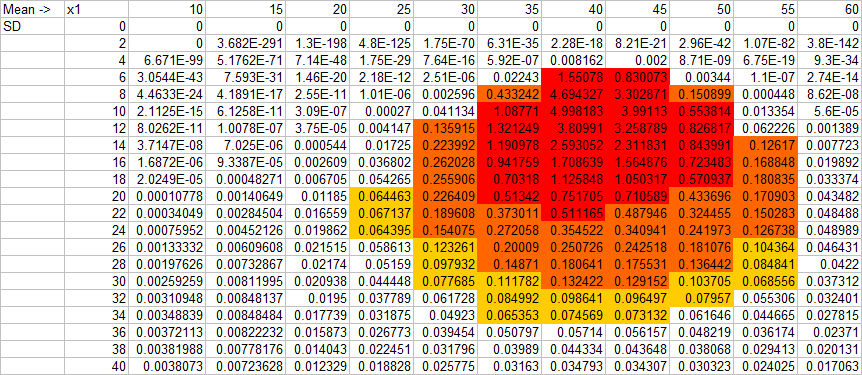See how much easier it is to read the axis values than using Excel's 3D surface chart.

To get a look at your results so far as new data are added you could show the normal-gamma distribution and then perhaps also the normal distribution for the highest point on that surface. Here is how you calculate the mean and standard deviation of the highest point:

```	mean = mu
standard deviation = SQRT(beta / (alpha - 1/2))
```

Alternatively, use the average value of the mean and the average value of the standard deviation, calculated like this:

```	mean = mu
standard deviation = SQRT(beta / alpha)
```

If you want to graph the normal distribution for a particular mean and standard deviation then use Excel's built in NORMDIST() function like this:

```	= NORMDIST(x, mean, stdev, FALSE)
```

## Focusing on the mean

This Bayesian recipe is a little more complicated than some others in this series because the frequency distribution has two parameters (the mean and standard deviation) rather than just one. The Bayesian calculations give a surface showing how likely you think each possible combination of mean and standard deviation is, given the data and your initial beliefs.

However, this is often just too much information! Usually it is the mean that we care about most. To understand how our beliefs about the true mean should be shaped we need to somehow sum all the possibilities over all the potential standard deviations. Fortunately, there is a well known distribution that does just that. It's called Student's T.

Student's T distribution has three parameters, called df (degrees of freedom), mu, and sigma. These can be calculated from the parameters of the normal-gamma using these simple formulae:

df = 2 × alpha

mu = mu       (yes that's right, it's the same number for both distributions

sigma = beta / (alpha × kappa)

Alternatively, copy and paste this code into the right module:

```Function t3_df(alpha)
t3_df = 2 * alpha
End Function

Function t3_mu(mu)
t3_mu = mu
End Function

Function t3_sigma(kappa, alpha, beta)
t3_sigma = beta / (alpha * kappa)
End Function
```

You can then use these new functions like this:

df = t3_df(alpha)

mu = t3_mu(mu)

sigma = t3_sigma(kappa, alpha, beta)

## Looking at results for the mean only

To view the evolving T distribution as more data are considered you need a suitable function to calculate points on it. Sadly, Excel's built in T distribution does not give probability densities and can't handle all three parameters. It only deals with the 'standard' T distribution. As always the solution is to paste in this code:

```Function t3_dens(x, df, mu, sigma)
' 3 parameter version of Student's T distibution, parameterised using the the scaling parameter
t3_dens = Exp(Application.GammaLn((df + 1) / 2) - Application.GammaLn(df / 2)) / _
(Application.Pi() * df * sigma * sigma) ^ 0.5 * _
(1 + 1 / df * ((x - mu) / sigma) ^ 2) ^ (-(df + 1) / 2)
End Function
```

With this in place you can make simple charts like this: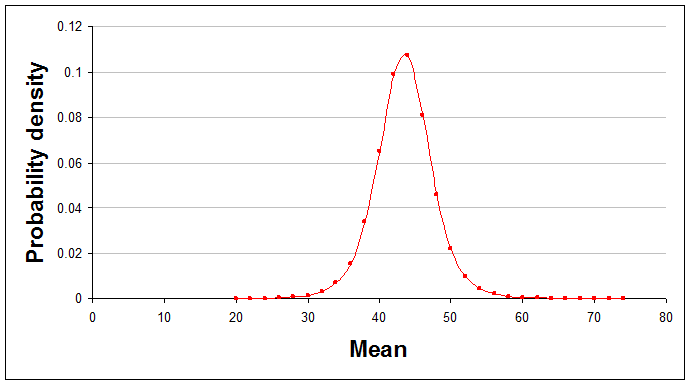As before, this shows the situation with all seven data points used.

## Summarising results so far for the mean only

All these graphs are comforting and give you a sense of how things are developing, but to summarise the situation precisely with numbers you need Excel to do some simple calculations. Here's how to do some different types of summary number, focusing only on the mean.

### Comparison with a threshold

Sometimes a helpful summary of your analysis is to say how likely it is that the mean of the normal distribution is above (or below) a stated threshold. (Notice that this is the mean of the true, underlying normal distribution, not a statement about the next data point or next sample.) For example, you might say something like "I'm 79% confident that the mean of the true distribution is less than 50."

To calculate numbers like this you need a function that Excel does not provide, so paste this into the appropriate module:

```Function t3_prob(x, df, mu, sigma)
' 3 parameter version of Student's T distribution, parameterised using the the scaling parameter
' get standardised T value
Dim t As Double
t = (x - mu) / sigma
If t >= 0 Then
t3_prob = 1 - Application.TDist(t, df, 1)
Else
t3_prob = Application.TDist(-t, df, 1)
End If
End Function
```

In the following formulae df, mu, and sigma are the parameters of the T distribution you have calculated and threshold is the threshold. Obviously you need to replace these with numbers or references in your spreadsheet.

To find the probability of the mean of the true normal distribution being below the threshold use:

```	= t3_prob(threshold, df, mu, sigma)
```

To find the probability of the mean of the true normal distribution being above the threshold use:

```	= 1 - t3_prob(threshold, df, mu, sigma)
```

The probability of the true mean being exactly equal to the threshold, when using the T distribution, is always zero.

### A central region

For most values of df, mu, and sigma that you will encounter the T distribution looks like a hill with just one peak. When it has this shape it makes sense to summarise the curve by stating a range of means that captures most of the area under the curve. This is the fat middle of the curve, rather than the thinner tails.

To find the probability that the truth lies between a lower and an upper threshold you have chosen use:

```	= t3_prob(upper, a, b) - t3_prob(lower, a, b)
```

To find the range that lies between the 10th and 90th percentile of the curve (i.e. an area that contains 80% of the probability) you need an inverse probability distribution so define your own function like this:

```Function t3_inv(p, df, mu, sigma)
Dim t As Double
If p < 0.5 Then
t = -Application.TInv(p * 2, df)
Else
t = Application.TInv((1 - p) * 2, df)
End If
t3_inv = mu + sigma * t
End Function
```

You can then calculate the percentiles with these formulae:

```	10 percentile level = t3_inv(0.1, df, mu, sigma)
90 percentile level = t3_inv(0.9, df, mu, sigma)
```

You could say, for example, something like "I am 80% confident that the true mean lies between 12 and 55."

Since the T distribution is symmetrical, the range between the 10th and 90th percentiles is also the narrowest range that contains 80% of the probability.

### Numbers to bet with

Having a distribution over all possible averages, or all possible combinations of average and standard deviation, is informative and helpful, especially for long term decision making and forecasting. It's much better than just having a best estimate of the true average. However, what do you do if you have to make a decision, or perhaps place a bet, on just the next period of time? For decision-making purposes it is generally better to hold on to the full distribution and use it in your decision model than to summarise into one number.

However, for some purposes a probability distribution for the next period is appropriate and useful. What you need is a probability distribution for the value of the next measurement that somehow combines all possible combination of mean and standard deviation (and their associated normal distributions) into one curve, but weighting each according to how likely it is.

The distribution you need is again the T distribution, with appropriate parameter values. The appropriate parameter values are similar to those for the mean-only curve, but slightly different:

df = 2 × alpha

mu = mu       (yes that's right, it's still the same number for both distributions

sigma' = beta × (kappa + 1) / (alpha × kappa)

If you want to know the probability of the next measurement being less than x then use:

```	= t3_prob(x, df, mu, sigma')
```

If you want to know the probability of the next measurement being more than x then use:

```	= 1 - t3_prob(x, df, mu, sigma')
```

If you want to know the probability of the next measurement being between low and high then use:

```	= t3_prob(high, df, mu, sigma') - t3_prob(low, df, mu, sigma')
```

### Comparing distributions

Another common requirement is to compare two or more distributions of measurements. There is a widely used statistical significance test for this, Student's t-test, so the technique below is a replacement for it.

This would be appropriate if you were studying two independent processes that each produced measurements that were normally distributed. You just apply the usual normal-gamma distribution technique described above to each of the processes to get df, mu, and sigma values for each. The resulting T distribution curves might look something like this: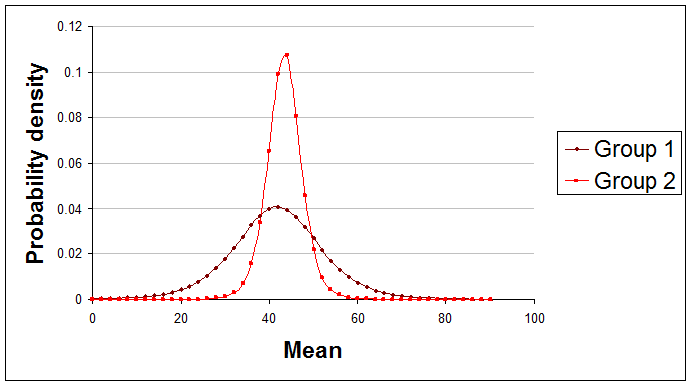Here are examples of useful summary statements about the difference between these two curves that you might make:

• "The true mean of Group 1 is only 46% likely to be greater than the true mean of Group 2."

• "There's only about 15% chance that Group 1's mean is at least 10 higher than Group 2's mean."

Sadly, Excel doesn't come even close to providing a function to do the comparison, but if you paste this code in as usual you can use this new function, t3_diff_prob, which calls t3_gen:

```Function t3_diff_prob(df1, mu1, sigma1, df2, mu2, sigma2, diff, runs)
Dim count As Long
count = 0
For i = 1 To runs
If t3_gen(Rnd(), Rnd(), df1, mu1, sigma1) > t3_gen(Rnd(), Rnd(), df2, mu2, sigma2) + diff Then
count = count + 1
End If
Next i
t3_diff_prob = count / runs
End Function

Function t3_gen(r1, r2, df, myu, sigma)
t3_gen = myu + sigma * Application.NormSInv(r1) / (Application.ChiInv(r2, df) / df) ^ 0.5
End Function
```

With two processes giving parameters df1, mu1, sigma1, df2, mu2, and sigma2 use:

```	= t3_diff_prob(df1, mu1, sigma1, df2, mu2, sigma2, diff, runs)
```

In this formula diff is the difference between the two means and runs is the number of iterations used to arrive at an answer. If you just want to know how likely it is that the true mean of one is bigger than the true mean of the other then use a diff of zero. The more iterations you use the more accurate the results, but the longer it takes. I suggest using 1,000 runs first to see what happens.

If you have more than two T distributions to compare then you can compare them in pairs and report your findings.

### Generating random numbers

You might for some reason want to generate random numbers that conform to the T distribution or the normal-gamma distribution. Generating numbers according to the T distribution is easy once you have defined the t3_inv() function (given above). To generate a number number each time the spreadsheet is recalculated use:

```	=t3_inv(RAND(), df, mu, sigma)
```

Where the normal-gamma distribution is what you want then you need to generate pairs of numbers each time, one for the mean and the other for the standard deviation. Generate the standard distribution number first, using the inverse gamma function:

```Function gamma_inv(r, a, b)
gamma_inv = Application.GammaInv(r, a, 1 / b)
End Function
```

Use it like this:

```	=gamma_inv(RAND(), alpha, beta)
```

The alpha and beta in this formula are the same as from the normal-gamma distribution you are using. Next, generate a number for the mean like this:

```	=NORMINV(RAND(), mu, SQRT(1 / (kappa * stdev)))
```

where stdev is the standard deviation generated in the first step.

# Sample sizes

With this approach to statistics there is no need to decide on your sample size in advance. You can just record each measurement or set of measurements, process the new data, and stop the moment your results are clear enough for your purposes.

Of course it can be helpful to have some idea in advance of how many measurements you might need to examine. To explore this just try possible sample sizes, sample averages, and sample variances and see what the normal-gamma or T distributions would look like.

# Limitations

As always, the examples of summary statements given above should really have been prefaced with the words "given the data used and the assumptions made," because we sometimes have a choice about which data to use, and because different results will arise if different assumptions are made.

# Finally

This is about as tricky as these recipes will get. That's because of there being two parameters for the underlying frequency distribution instead of just one.

Hundreds of people receive notification of new publications every month. They include company directors, heads of finance, of internal audit, of risk management, and of internal control, professors, and other influential authors and researchers.